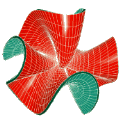Sfb 288 Differential Geometry and Quantum PhysicsGeodesics and waveson piecewise linear surfacesGeodesic curves are a fundamental concept in geodesy and mathematics to generalize the idea of a "straight line" to curved surfaces and general manifolds. The straight line property makes them a helpful concept in numerical methods on arbitrary surfaces and especially discrete surfaces.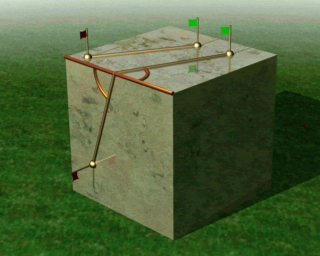On a piecewise linear surface a discrete geodesic across an edge must have equal angle on both sides.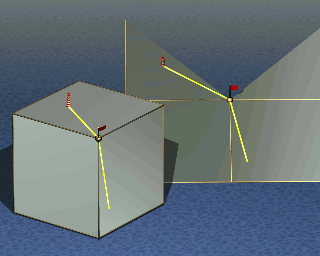A straightest curve through a vertex of the surface must bisect its total vertex angle.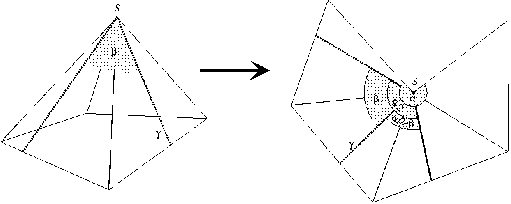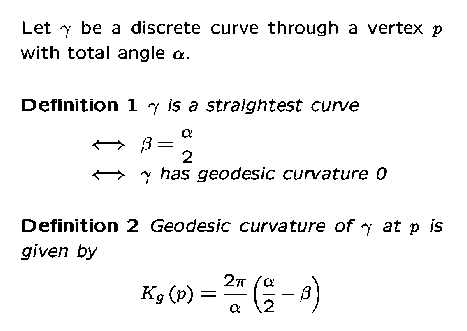For the numerical integration of a vector field v on a curved surface with e.g. an Euler Method geodesics may be used in the basic step as follows: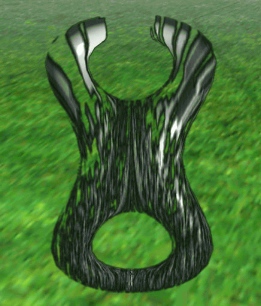From an initial point p proceed a distance h along the geodesic with initial point p and direction v(p). This avoids ambiguous projection operations needed in conventional methods.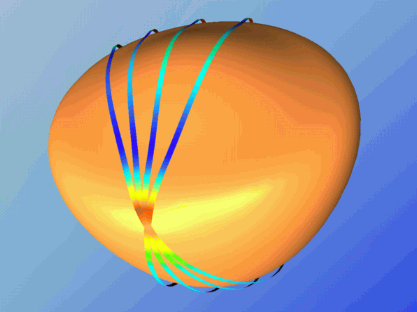A highly discretised Zoll's Surface with regions of strict negative curvature. The four discrete geodesics are almost closed. The curvature of the surface determines its color.

An Example: Discrete Geodesics on Zoll's Surfaces
Zoll's surfaces [O.Zoll,1903] have the property that all their geodesics are simply closed and have same length. They come in a family of rotational surfaces whose meridians are given as solutions of an ODE. It is remarkable that the family contains surfaces which have locally strict negative gaussian curvature. The closedness property of geodesics on a Zoll's suface makes them suitable candidates for testing the concept of discrete geodesics.Author: Konrad Polthier, Markus Schmies

Copyright © 1999 Sfb 288, Mathematics 8-5, Strasse des 17 Juni 136, TU-Berlin, 10623 Berlin